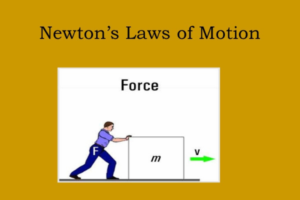Thu. Nov 30th, 2023

# Newton’s Laws of Motion-Know Everything About It

## Understanding the concept of Newton’s laws of Motion

It’s a well observed fact that an isolated object at rest does not start moving on their own. Rather it requires effort in order to move the object. For example, when we push or pull a table, we are exerting a force on the table.

Inertia is the inherent property of objects to remain at rest unless acted upon by a force. Also, the property of a body to keep moving with constant velocity in the absence of any force acting on it is its inertia. Firstly, it was Galileo who discovered the Law of Inertia. Bodies moving with uniform velocity would maintain this state of motion forever in the absence of forces acting upon them.Later on, the basis of Galileo’s findings, Issac Newton formulated his three laws of motion which became the foundations of mechanics.

### Newton’s First Law of Motion-

Newton’s first law is a reformulation of Galileo’s law of inertia. First of all, let’s try to understand the concept of inertia here. So, the property of a body to continue in its state of rest or that of uniform motion in a straight line in the absence of external force is called inertia. And Newton’s law states that- every body continues in its state of rest or of uniform motion in a straight line unless compelled by some external force to act otherwise.

Momentum- is the product of mass (M) of a body and its velocity (V). thus,

Momentum = Mass Velocity

### Newton’s Second Law of Motion-

It deals with the rate of change of momentum of an object. Newton’s second law states that the rate of change of momentum of an object is directly proportional to the force acting on it and takes place in the direction in which the force acts.

Newton’s second law of motion gives-

1. Expression of force
2. The direction of force

Equation- F=m x a, where m=mass, a=acceleration.

Force= Change in momentum/ time

Units of force-

1. SI unit of force = Newton

1 Newton= 1kg m s-2

CGS unit force = Dyne

1 dyne= 1g cm s-2

1. Gravitational unit of force

SI system= 1 k gf= 9.8N

CGS system= 1gf= 980 dyne

Relation between newton and dyne

1 newton= 105 dyne

### Newton’s Third of Motion-

It states that “to every action there is always an equal and opposite reaction.” It may be noted that action and reaction which occur in pairs act on different bodies. If they are acted on the same body, the resultant force would be zero and there could never be accelerated motion.